Question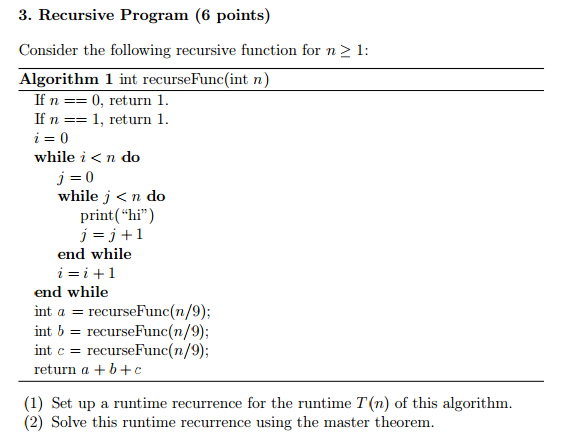We need at least 10 more requests to produce the answer.

0 / 10 have requested this problem solution

The more requests, the faster the answer.

All students who have requested the answer will be notified once they are available.

#### Earn Coins

Coins can be redeemed for fabulous gifts.

Similar Homework Help Questions
• ### please also explain how the answer came about if possible b) Base 7. Master Theorem (3...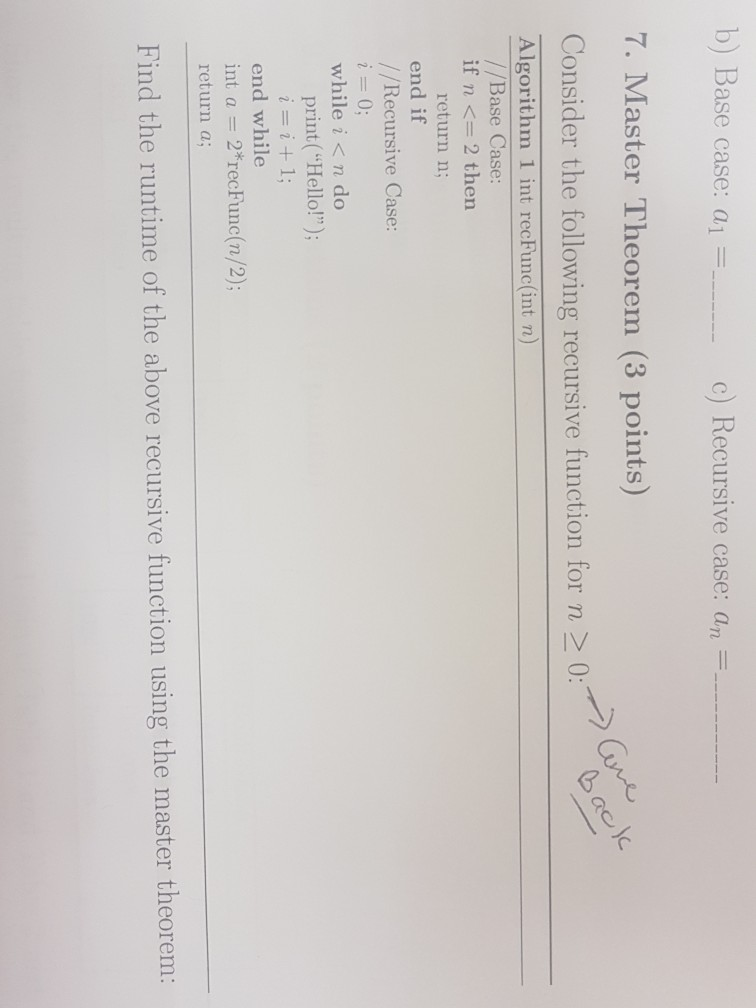please also explain how the answer came about if possible b) Base 7. Master Theorem (3 points) Consider the following recursive function for n >0 case: a| c) Recursive case: an Algorithm 1 int recFunc(int n) //Base Case if n <= 2 then return n; end if / /Recursive Case: while i< n do print("Hello!") end while int a 2*recFunc(n/2); return a; Find the runtime of the above recursive function using the master theorem

• ### PROBLEM 1 (24 points): For each of the recursive functions below and on the next page,...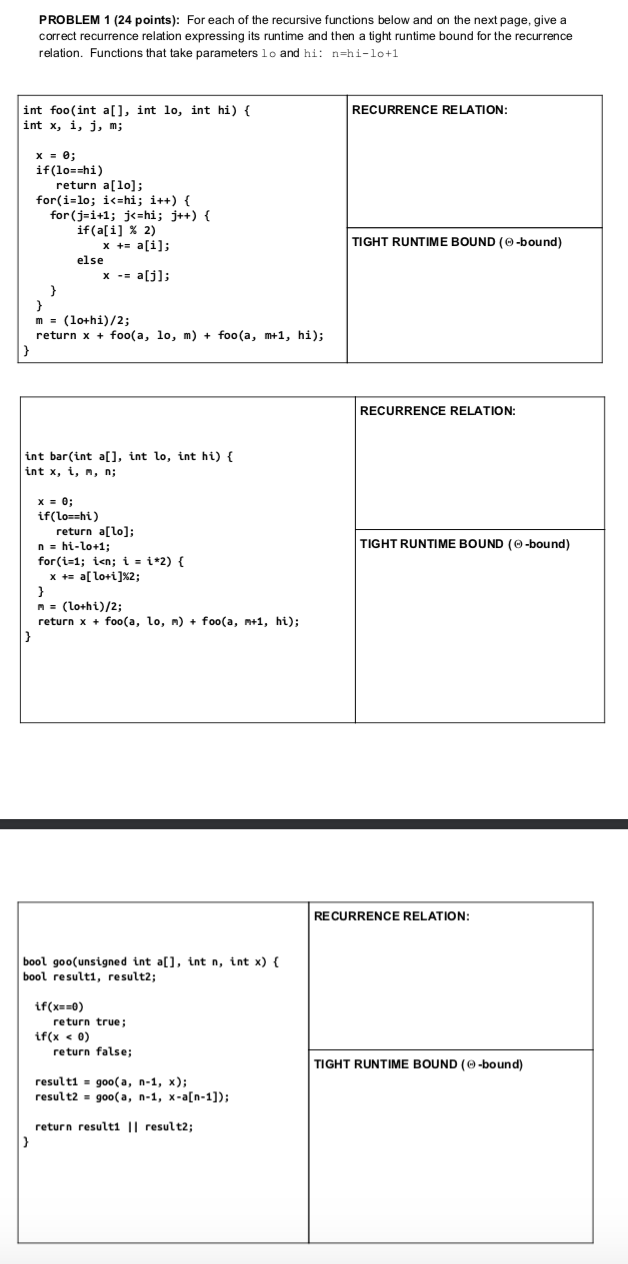PROBLEM 1 (24 points): For each of the recursive functions below and on the next page, give a correct recurrence relation expressing its runtime and then a tight runtime bound for the recurrence relation. Functions that take parameters lo and hi: n=hi-lo+1 RECURRENCE RELATION: int foo(int a[], int lo, int hi) { int x, i, j, m; X = 0; if(lo==hi) return a; for(i=lo; i<=hi; i++) { for(j=i+1; j<=hi; j++) { if(a[i] % 2) x += a[i]; else x -=...

• ### 3) [16 points total] Consider the following algorithm int SillyCalc (int n) int i; int Num, answer; if (n <=...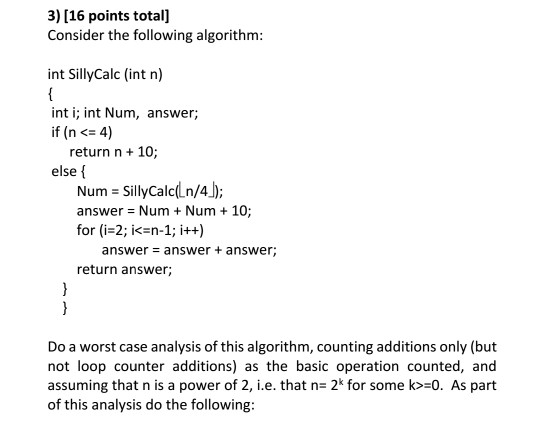3) [16 points total] Consider the following algorithm int SillyCalc (int n) int i; int Num, answer; if (n <= 4) return n 10; else { Num-SillyCalcl n/4) answer = Num + Num + 10; for (i-2; i<-n-1; ++) answer- answer+ answer; return answer; Do a worst case analysis of this algorithm, counting additions only (but not loop counter additions) as the basic operation counted, and assuming that n is a power of 2, i.e. that n- 2* for some...

• ### Consider the following recursive algorithm for computing the sum of the first n cubes: S(n) =...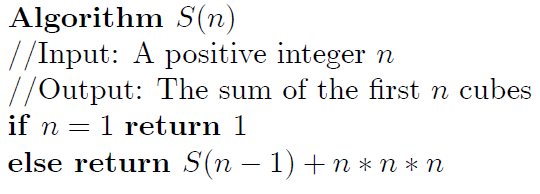Consider the following recursive algorithm for computing the sum of the first n cubes: S(n) = 13 + 23 + … + n3. (a) Set up a recurrence relation for the number of multiplications made by this algorithm. (b) Provide an initial condition for the recurrence relation you develop at the question (a). (c) Solve the recurrence relation of the question (a) and present the time complexity as described at the question number 1. Algorithm S n) Input: A positive...

• ### 2. Consider the function george (int n) computed by the following recursive C++ code. int george ...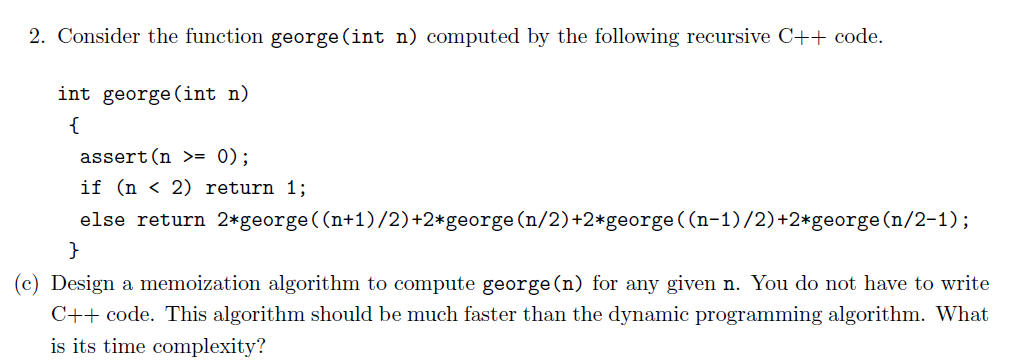2. Consider the function george (int n) computed by the following recursive C++ code. int george (int n) assert (n >= 0) if (n < 2) return 1; else return 2*george ((n+1)/2)+2*george (n/2)+2 george((n-1)/2)+2*george (n/2-1); (c) Design a memoization algorithm to compute george(n) for any given n. You do not have to write C++ code. This algorithm should be much faster than the dynamic programming algorithm. What is its time complexity? 2. Consider the function george (int n) computed by...

• ### 3) [16 points totall Consider the following algorithm: int SillyCalc (int n) { int i; int Num, answer; if (n &lt...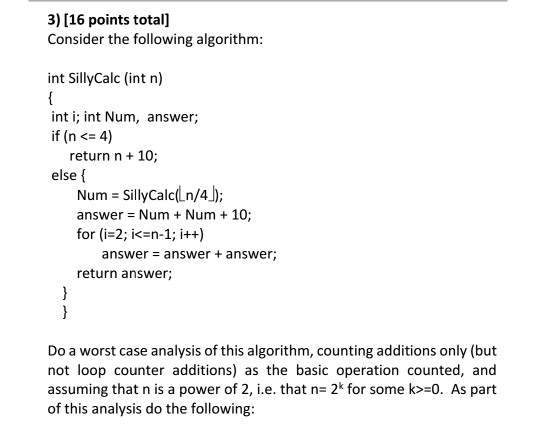3) [16 points totall Consider the following algorithm: int SillyCalc (int n) { int i; int Num, answer; if (n < 4) 10; return n+ else f SillyCalc(Ln/4) answer Num Num 10 for (i-2; i<=n-1; i++) Num + = answer + answer; answer return answer } Do a worst case analysis of this algorithm, counting additions only (but not loop counter additions) as the basic operation counted, and assuming that n is a power of 2, i.e. that n- 2*...

• ### 3. Consider the mystery method given. public static int mystery ( int n) [ if (n...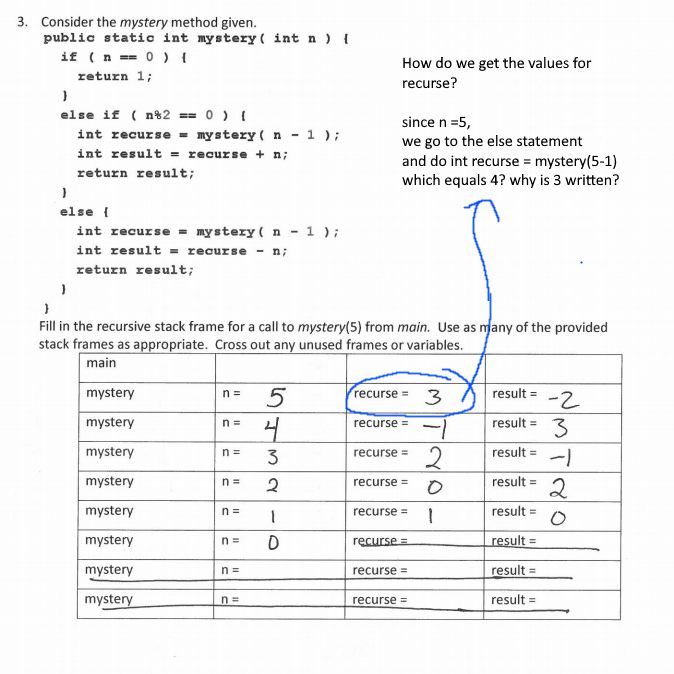3. Consider the mystery method given. public static int mystery ( int n) [ if (n == 0 ) { return 1; How do we get the values for recurse? else if (n%2 == 0 ) { int recurse = mystery ( n - 1); int result = recurse + n; return result; since n =5, we go to the else statement and do int recurse = mystery(5-1) which equals 4? why is 3 written? else { int recurse =...

• ### 6. Consider the following algorithm, where P is an array containing random numbers. The function swap(v1,v2)...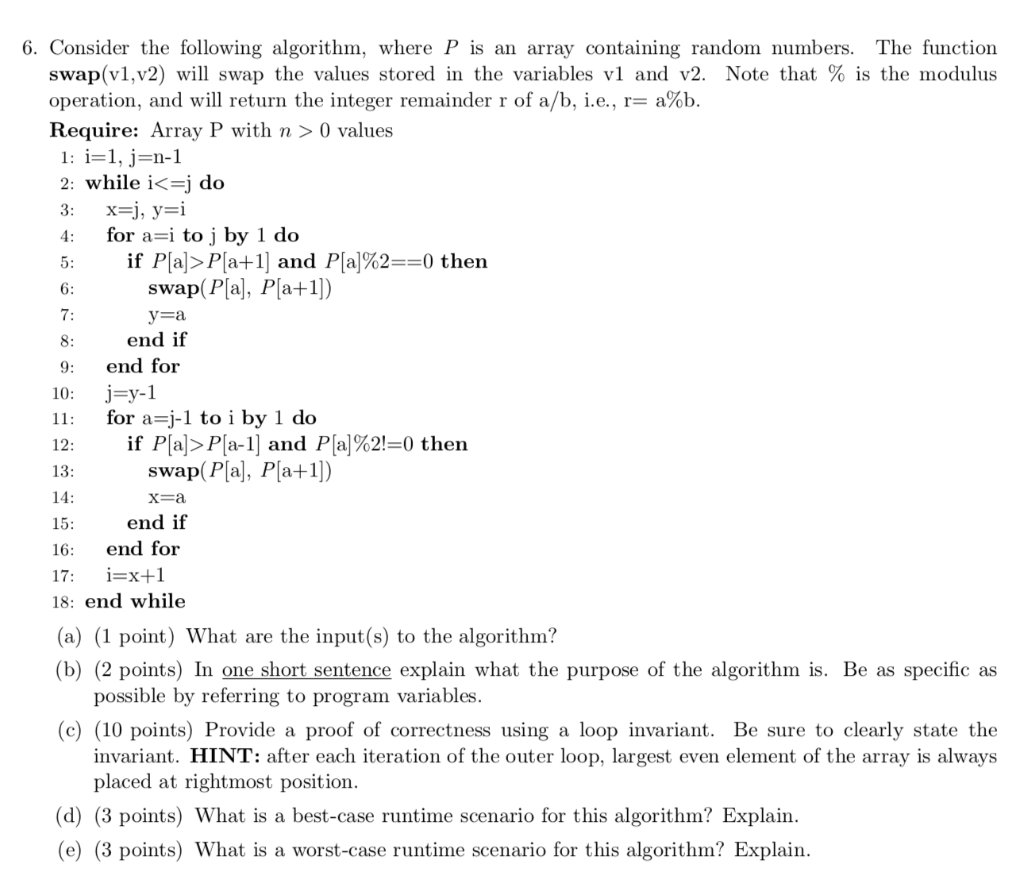6. Consider the following algorithm, where P is an array containing random numbers. The function swap(v1,v2) will swap the values stored in the variables v1 and v2. Note that % is the modulus operation, and will return the integer remainder r of a/b, i.e., r-a%b Require: Array P with n > 0 values 1: i-1, j-n-l 2: while i<=j do for a=i to j by i do 4: 5: 6: 7: if Pla>Pat 11 and Pla]%2--0 then swap(Plal, Pla+1l) end...

• ### 7. Consider the following proposed sorting algorithm supersort (int n, int start, int end, keytype SI1)1...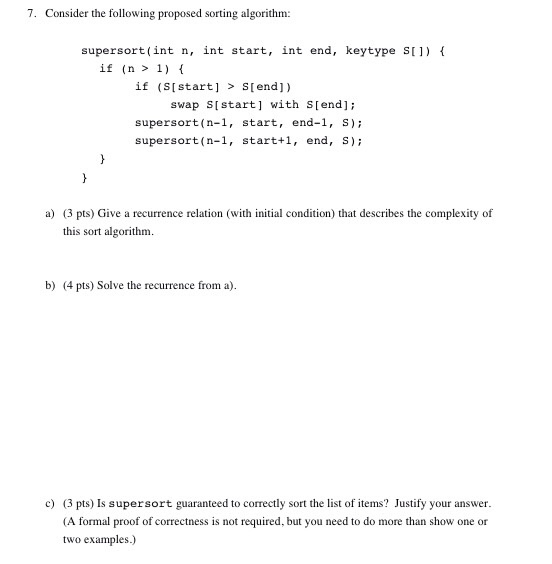7. Consider the following proposed sorting algorithm supersort (int n, int start, int end, keytype SI1)1 if(n > 1) { if (SIstart] > S[end]) swap SIstart] with Stend]; supersort(n-l, start, end-1, s) supersort (n-1, start+, end, S) a) 3 pts) Give a recurrence relation (with initial condition) that describes the complexity of this sort algorithm b) (4 pts) Solve the recurrence froma) c) (3 pts) Is supersort guaranteed to correctly sort the list of items? Justify your answer. (A formal...

• ### The following algorithm (Rosen pg. 363) is a recursive version of linear search, which has access...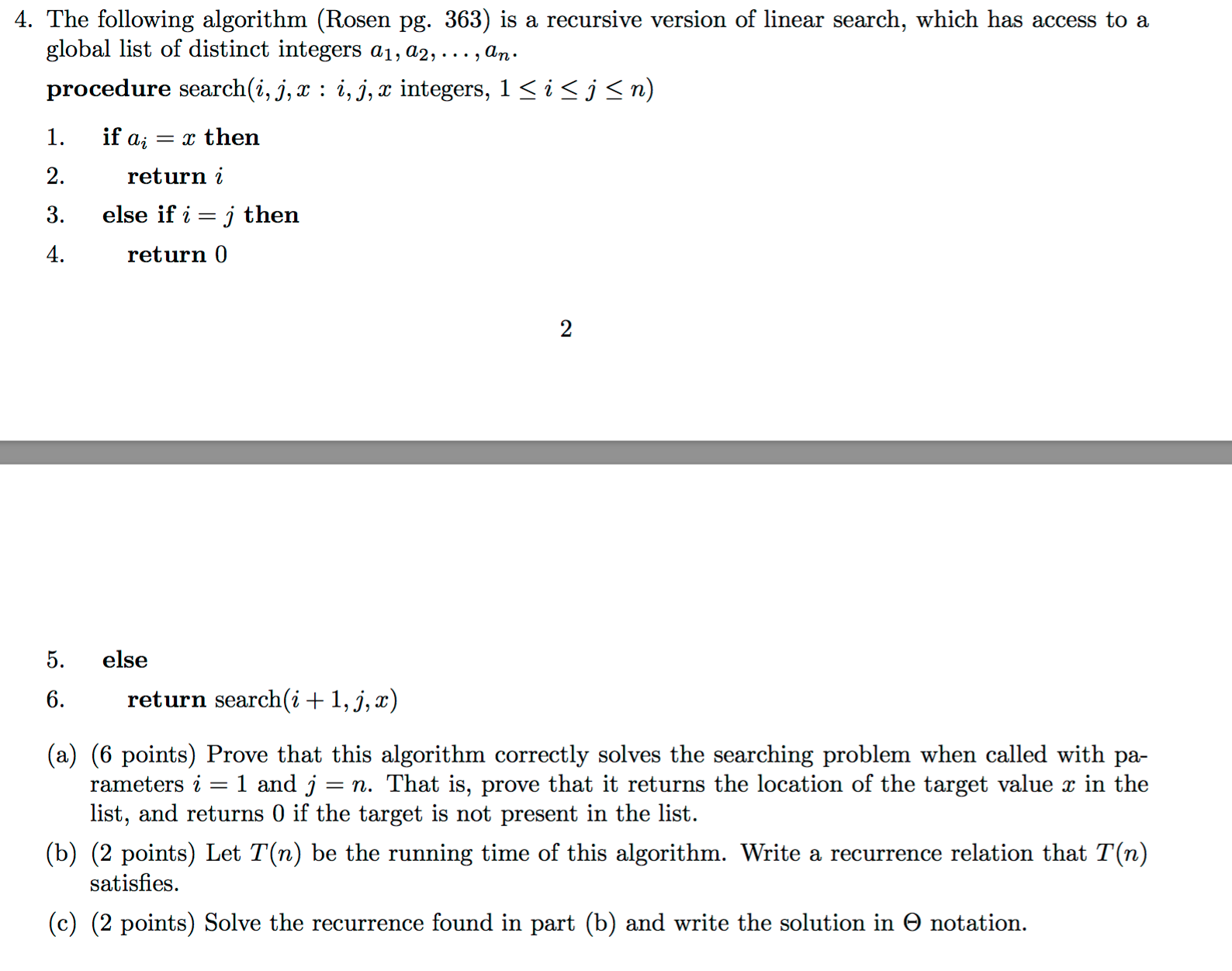The following algorithm (Rosen pg. 363) is a recursive version of linear search, which has access to a global list of distinct integers a_1, a_2,..., a_n. procedure search(i, j, x : i,j, x integers, 1 < i < j < n) if a_i = x then return i else if i = j then 4. return 0 else return search(i + 1, j, x) Prove that this algorithm correctly solves the searching problem when called with parameters i = 1...